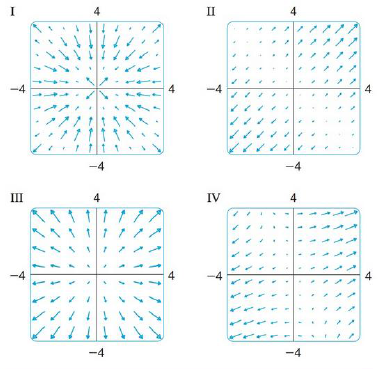Chapter 16.1, Problem 31E

Chapter
Section
Textbook Problem

Match the functions f with the plots of their gradient vector fields labeled I-IV. Give reasons for your choices.31. f(x, y) = (x + y)2To determine

To match: The function f(x,y)=(x+y)2 with the plots labeled as I-IV.

Explanation

Given data:

f(x,y)=(x+y)2 (1)

Formula used:

Write the expression for gradient vector field of a two dimensional vector.

f(x,y)=fxi+fyj (2)

Write the required differentiation formulae with respect to x as follows.

x(x2)=2xx(2xy)=2yx(y2)=0

Write the required differentiation formulae with respect to y as follows.

y(y2)=2yy(2xy)=2xy(x2)=0

Rearrange equation (1) as follows.

f(x,y)=(x+y)2=x2+y2+2xy

Differentiate the term x2+y2+2xy with respect to x .

x(x2+y2+2xy)=x(x2)+x(y2)+x(2xy)=2x+0+2y=2x+2y=2(x+y)

Differentiate the term x2+y2+2xy with respect to y

Still sussing out bartleby?

Check out a sample textbook solution.

See a sample solution

The Solution to Your Study Problems

Bartleby provides explanations to thousands of textbook problems written by our experts, many with advanced degrees!

Get Started

In Problems 15-18, find the second partials.

Mathematical Applications for the Management, Life, and Social Sciences

Find the prime factorization of 54.

Elementary Technical Mathematics

limxx3ex2

Calculus: Early Transcendental Functions (MindTap Course List)

For what values of p does the series converge?

Study Guide for Stewart's Single Variable Calculus: Early Transcendentals, 8th